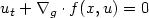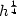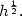Hostname: page-component-7d8f8d645b-dvxft Total loading time: 0 Render date: 2023-05-29T11:37:45.726Z Has data issue: false Feature Flags: { "useRatesEcommerce": true } hasContentIssue false

# A convergence result for finite volume schemes on Riemannian manifolds

Published online by Cambridge University Press:  12 June 2009

## Abstract

This paper studies a family of finite volume schemes for the hyperbolic scalar conservation law $u_t +\nabla_g \cdot f(x,u)=0$on a closed Riemannian manifold M. For an initial value in BV(M) we will show that these schemes converge with a $h^{\frac{1}{4}}$convergence rate towards the entropy solution. When M is 1-dimensional the schemes are TVD and we will show that this improves the convergence rate to $h^{\frac{1}{2}}.$Type
Research Article
Information

## Access options

Get access to the full version of this content by using one of the access options below. (Log in options will check for institutional or personal access. Content may require purchase if you do not have access.)

## References

Amorim, P., Ben-Artzi, M. and LeFloch, P.G., Hyperbolic conservation laws on manifolds: total variation estimates and the finite volume method. Methods Appl. Anal. 12 (2005) 291323.
Ben-Artzi, M. and LeFloch, P.G., Well-posedness theory for geometry compatible hyperbolic conservation laws on manifolds. Ann. H. Poincaré Anal. Non Linéaire 24 (2007) 9891008. CrossRef
Calhoun, D.A., Helzel, C. and LeVeque, R.J., Logically rectangular grids and finite volume methods for PDEs in circular and spherical domains. SIAM Rev. 50 (2008) 723752. Available at http://www.amath.washington.edu/~rjl/pubs/circles. CrossRef
Cho, J.Y.-K. and Polvani, L.M., The emergence of jets and vortices in freely evolving, shallow-water turbulence on a sphere. Phys. Fluids 8 (1996) 15311552. CrossRef
Dikpati, M. and Gilman, P.A., A “shallow-water” theory for the sun's active longitudes. Astrophys. J. Lett. 635 (2005) L193L196. CrossRef
M.P. do Carmo, Riemannian geometry, Mathematics: Theory & Applications. Birkhäuser Boston Inc., Boston, USA (1992).
Eymard, R., Gallouët, T., Ghilani, M. and Herbin, R., Error estimates for the approximate solutions of a nonlinear hyperbolic equation given by finite volume schemes. IMA J. Numer. Anal. 18 (1998) 563594. CrossRef
Font, J.A., Numerical hydrodynamics and magnetohydrodynamics in general relativity. Living Rev. Relativ. 11 (2008) 7. URL (cited on June 8, 2009): http://www.livingreviews.org/lrr-2008-7. CrossRef
Gilman, P.A., Magnetohydrodynamic “shallow-water” equations for the solar tachocline. Astrophys. J. Lett. 544 (2000) L79L82. CrossRef
Giraldo, F.X., Lagrange-Galerkin methods on spherical geodesic grids. J. Comput. Phys. 136 (1997) 197213. CrossRef
Giraldo, F.X., High-order triangle-based discontinuous Galerkin methods for hyperbolic equations on a rotating sphere. J. Comput. Phys. 214 (2006) 447465. CrossRef
Iacono, R., Struglia, M.V. and Ronchi, C., Spontaneous formation of equatorial jets in freely decaying shallow water turbulence. Phys. Fluids 11 (1999) 12721274. CrossRef
J. Jost, Riemannian Geometry and Geometric Analysis. Springer Universitext, Springer (2002).
Lanser, D., Blom, J.G. and Verwer, J.G., Spatial discretization of the shallow water equations in spherical geometry using osher's scheme. J. Comput. Phys. 165 (2000) 542565. CrossRef
Martíand, J.M. Müller, E., Numerical hydrodynamics in special relativity. Living Rev. Relativ. 6 (2003) 7. URL (cited on June 8, 2009): http://www.livingreviews.org/lrr-2003-7.
Miranda, M.J., Pallara, D., Paronetto, F. and Preunkert, M., Heat semigroup and functions of bounded variation on Riemannian manifolds. J. reine angew. Math. 613 (2007) 99119.
Rancic, M., Purser, R.J. and Mesinger, F., A global shallow-water model using an expanded spherical cube: Gnomonic versus conformal coordinates. Q. J. R. Meteorolog. Soc. 122 (1996) 959982. CrossRef
Ronchi, C., Iacono, R. and Paolucci, P.S., The cubed sphere: A new method for the solution of partial differential equations in spherical geometry. J. Comput. Phys. 124 (1996) 93114. CrossRef
Rossmanith, J.A., A wave propagation algorithm for hyperbolic systems on the sphere. J. Comput. Phys. 213 (2006) 629658. CrossRef
Rossmanith, J.A., Bale, D.S. and LeVeque, R.J., A wave propagation algorithm for hyperbolic systems on curved manifolds. J. Comput. Phys. 199 (2004) 631662. CrossRef
Schecter, D.A., Boyd, J.F. and Gilman, P.A., “Shallow-water” magnetohydrodynamic waves in the solar tachocline. Astrophys. J. Lett. 551 (2001) L185L188. CrossRef
Tsukahara, Y., Nakaso, N., Cho, H. and Yamanaka, K., Observation of diffraction-free propagation of surface acoustic waves around a homogeneous isotropic solid sphere. Appl. Phys. Lett. 77 (2000) 29262928. CrossRef